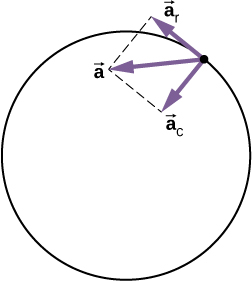# Centripetal force and change of the tangential velocity

I'm studying physics in the fifths semester and I'm still confused by some aspects of circular motion. So, I understand that the centripetal force changes the direction of the velocity, whereas the speed stays the same. But when the direction of the velocity changes, what causes the change of the tangential velocity at the uppermost point of the vertical circle ( since the tangential velocity has its direction in horizontal as well as in perpendicular direction as in the picture below )? Because obviously it has to change but the only applied force is the centripetal force, which does not "interact" with the tangential velocity.• It looks like you are essentially saying "I understand the centripetal force changes the direction of velocity, but what causes the change in the direction of the velocity?" You recognize the tangential velocity only changes direction (for uniform circular motion), and you recognize this is caused by the centripetal component of the net force. So I'm not sure where the disconnect is. Aug 31, 2020 at 11:59
• Yeah, you're right, I got it wrong. I mean, the centripetal force accelerates the body and therefore gives it new velocity - component perpendicular to the tangential velocity. But since the speed stays the same, the tangential velocity must decrease. So does the centripetal force "take" a piece of the tangential velocity and "give" it to the component perpendicular to it? For me it doesn't make sense, because the force is also perpendicular to the tangential velocity and therefore should not influence it. Aug 31, 2020 at 12:07
• Why do you think if the speed stays the same the tangential velocity decreases? In circular motion the velocity is only tangential, so constant speed means constant tangential velocity magnitude. Aug 31, 2020 at 12:11
• @Bababa Yes why should the tangential velocity change ? There is no other velocity than the tangential velocity otherwise if it existed the body will not fly off tangentially if the force is removed rather would take the path obtained by the resultant of the two velocities. Aug 31, 2020 at 12:15
• Yeah both of you are absolutely right. Well, consider a circle and a particle on top of the circle. Then the vector of the velocity is parallel to the ground. Just a moment later, the velocity has a different angle. So when we brake the vector down in two components, namely one, which is parallel to the ground and one, perpendicular to it, the previous component parallel to the ground decreases. But, since the centripetal force is perpendicular to it, it should not change it. So, when I mentiones "tangential velocity", I meant the component parallel to the ground, I'm sorry! Aug 31, 2020 at 12:28

First some clarification. In circular motion, velocity is actually the same as tangential velocity, or in other words, there is no radial component of the velocity (otherwise it wouldn't describe a circle).

Now, a force produces an acceleration since $$\vec{F}=m ~\vec{a}$$, and an acceleration is a change of velocity in a given time interval. So the centripetal force does "interact" with the velocity (thus with the tangential velocity) because this force produces an acceleration $$\vec{a}= \vec{F}/m$$ which is $$\vec{a} = \Delta \vec{v}/ \Delta t = (\vec{v}_2-\vec{v}_1)/\Delta t$$.

$$\vec{v}_1$$ and $$\vec{v}_2$$ are vectors, so you have to subtract one from the other in vector fashion. Their speed does not change, but their direction does, so here the angle $$\alpha$$ and $$\Delta t$$ will enter into the equation.
Although space is 3-D, you might notice that this motion takes place in a plane, so you can ignore one of the 3 space coordinates.

If you are talking about a mass moving in a vertical circle on the end of a string, there are two forces acting: the tension in the string and gravity. At the top of the circle, both are acting down and the tangential speed is not changing. (The sum of the two produces the centripetal acceleration.) At any other point, gravity has a tangential component. The tangential speed increases as the mass is coming down and decreases as it goes up.

• No, I just thought of a isolated system, no forces at all (except the centripetal force). And now I'm wondering what causes the change in the horizontal component of vector 1? Aug 31, 2020 at 15:14
• If the object is moving in a horizontal circle, the change in the velocity vector during a very short interval of time is caused by the centripetal force and is directed toward the center of the circle. Keep in mind that the Δv on your vector diagram represents a vector sum of many small Δv's which vary in direction. Aug 31, 2020 at 16:25
• just consider any motion on a plane along a line with constant velocity , than give it a small push perpendicular to the line, what will happen ? Aug 31, 2020 at 17:04
• If the only force is the centripetal force, then there is no change in the tangential speed. Aug 31, 2020 at 17:12

In general for any particle in circular motion, the net acceleration of the particle is

$$a_{net} = \sqrt[]{a_r^2 + a_c^2}$$It can be seen that net acceleration is little off center. For the particles whose speeds do change some part of this acceleration modifies the tangential velocity, and other changed its direction.

Consider a simple example, a person is swinging a bob tied to a string horizontally. A careful look at his hand shows that his hand ( that is producing overall acceleration for the motion) is pull in the direction of $$a_{net}$$.

For cases where the circular motion is uniform, only tangential acceleration remains that is changing its direction.

$$a_{net} = \sqrt[]{0^2 + a_c^2} = a_c$$